﻿ Square channel with a curved section - XSim

# Square channel with a curved section

OpenFOAM 4.x

## Case directory

\$FOAM_TUTORIALS/compressible/rhoSimpleFoam/squareBend

## Summary

We calculate the air flow through a square channel of 50 mm × 50 mm with a curved section.

The air flows in from the region "inlet" at 0.5 kg/s and out from the region "outlet". The walls of the flow channel are set to no-slip wall.Model geometry

The standard k-ε model is used for the turbulence model.

The meshes are as follows, and the number of mesh is 112400.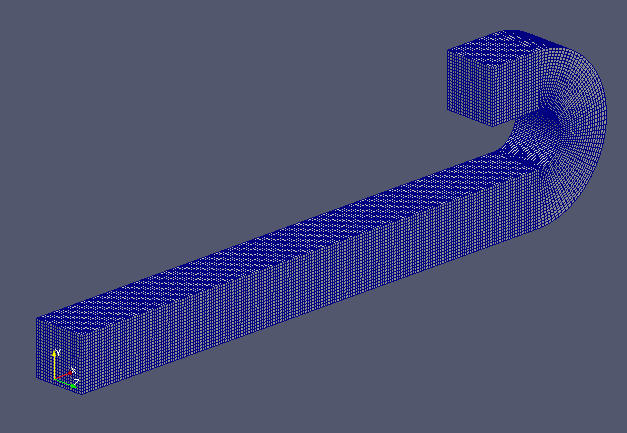Mesh (entire)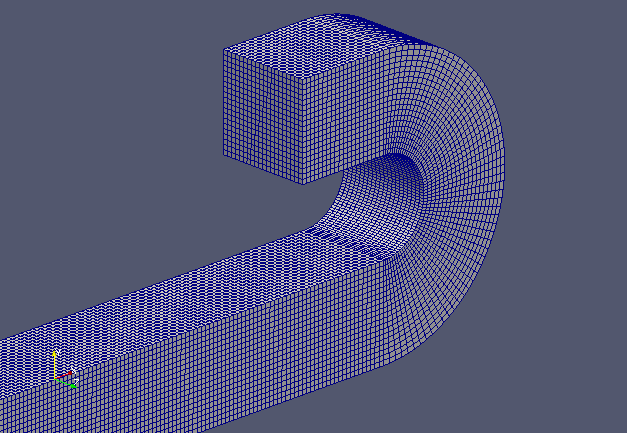Mesh (curved section)

If you want to visualize turbulent energy and turbulent dissipation rate, check "k" and "epsilon" in the "Properties" tab in ParaView.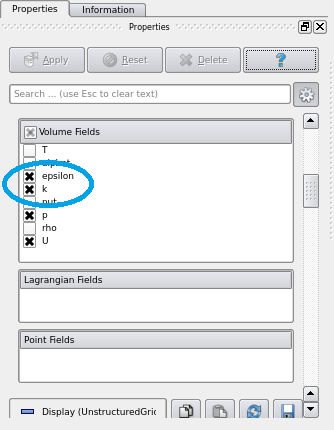Check "k" and "epsilon" in "Properties" tab

The calculation result is as follows.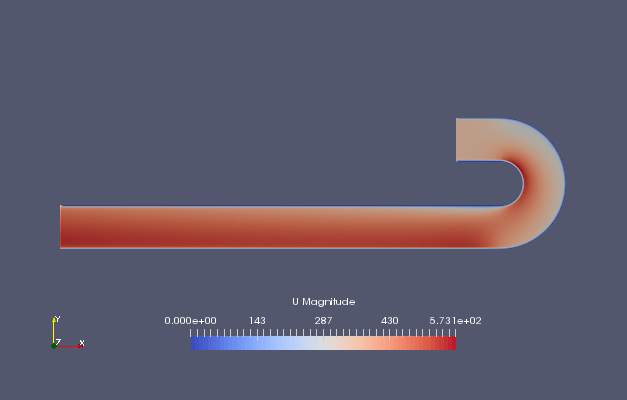Flow velocity (U)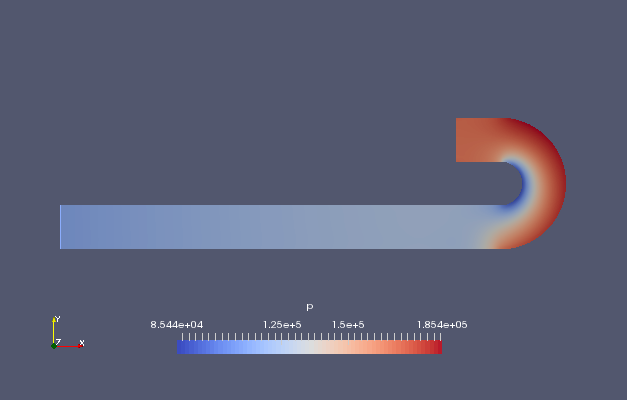Pressure (p)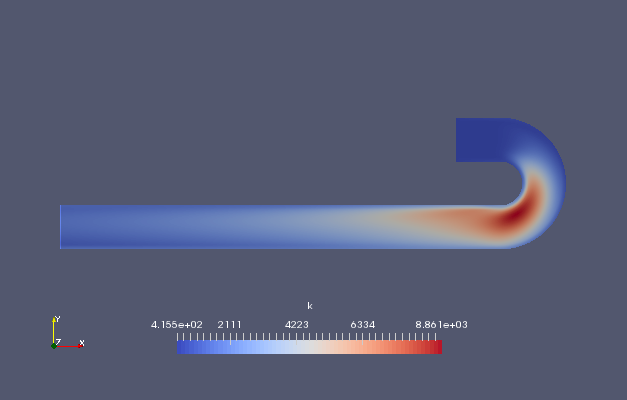Turbulent energy (k)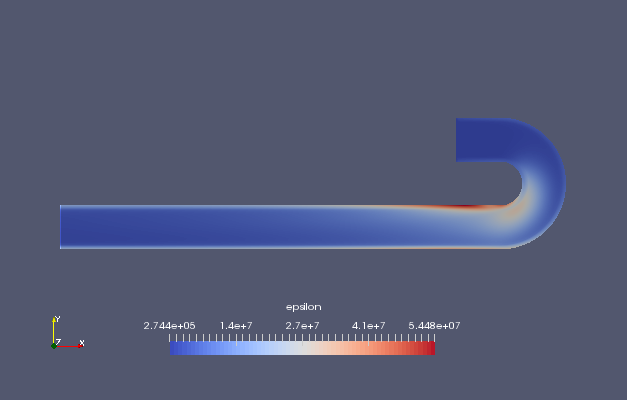Turbulent dissipation rate (epsilon)

We can see that the pressure drops significantly at the curved section.

## Commands

cp -r \$FOAM_TUTORIALS/compressible/rhoSimpleFoam/squareBend squareBend
cd squareBend

blockMesh
decomposePar
mpirun -np 8 rhoSimpleFoam -parallel
reconstructParMesh -mergeTol 1e-06
reconstructPar

paraFoam

## Calculation time

20.68 seconds *8 parallel、Inter(R) Core(TM) i7-8700 CPU @ 3.20GHz 3.19GHz